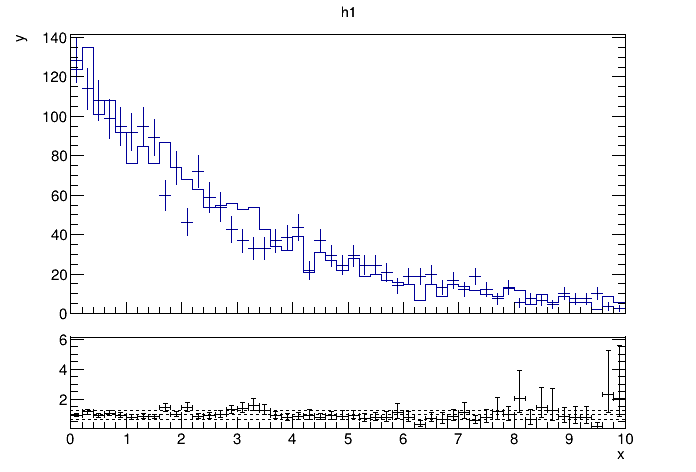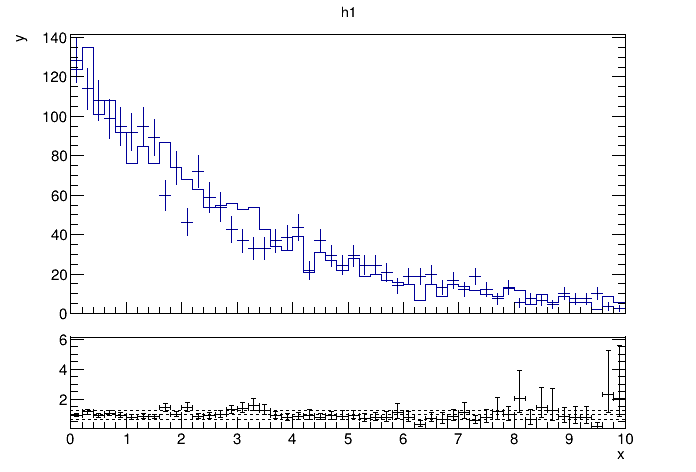# Ratioplot 1¶

Example creating a simple ratio plot of two histograms using the pois division option. Two histograms are set up and filled with random numbers. The constructor of TRatioPlot takes the to histograms, name and title for the object, drawing options for the histograms (hist and E in this case) and a drawing option for the output graph. Inspired by the tutorial of Paul Gessinger.

Author: Alberto Ferro
This notebook tutorial was automatically generated with ROOTBOOK-izer from the macro found in the ROOT repository on Thursday, May 19, 2022 at 08:14 AM.

In :
import ROOT

ROOT.gStyle.SetOptStat(0)

c1 = ROOT.TCanvas("c1", "A ratio example")
h1 = ROOT.TH1D("h1", "h1", 50, 0, 10)
h2 = ROOT.TH1D("h2", "h2", 50, 0, 10)
f1 = ROOT.TF1("f1", "exp(- x/ )")
f1.SetParameter(0,3)

h1.FillRandom("f1",1900)
h2.FillRandom("f1", 2000)
h1.Sumw2()
h2.Scale(1.9/2.)

h1.GetXaxis().SetTitle("x")
h1.GetYaxis().SetTitle("y")

rp = ROOT.TRatioPlot(h1,h2)

c1.SetTicks(0,1)
rp.GetLowYaxis().SetNdivisions(505)
c1.Update()
c1.Draw()
rp.Draw()

Welcome to JupyROOT 6.27/01Draw all canvases

In :
from ROOT import gROOT
gROOT.GetListOfCanvases().Draw()